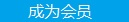您可以捐助，支持我们的公益事业。 1元 10元 50元 认证码：必填求知 文章 文库 Lib 视频 iProcess 课程 角色 咨询 工具 讲座 Modeler Code要资料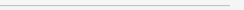订阅捐助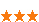369 次浏览     评价： 好 中 差
2019-5-5

 编辑推荐: 本文来自于博客园，本文主要使用机器学习算法来将个体机器学习器的结果结合在一起，这个方法就是Stacking，希望对您的学习有所帮助。

Ensemble learning 中文名叫做集成学习，它并不是一个单独的机器学习算法，而是将很多的机器学习算法结合在一起，我们把组成集成学习的算法叫做“个体学习器”。在集成学习器当中，个体学习器都相同，那么这些个体学习器可以叫做“基学习器”。

Stacking 的基本思想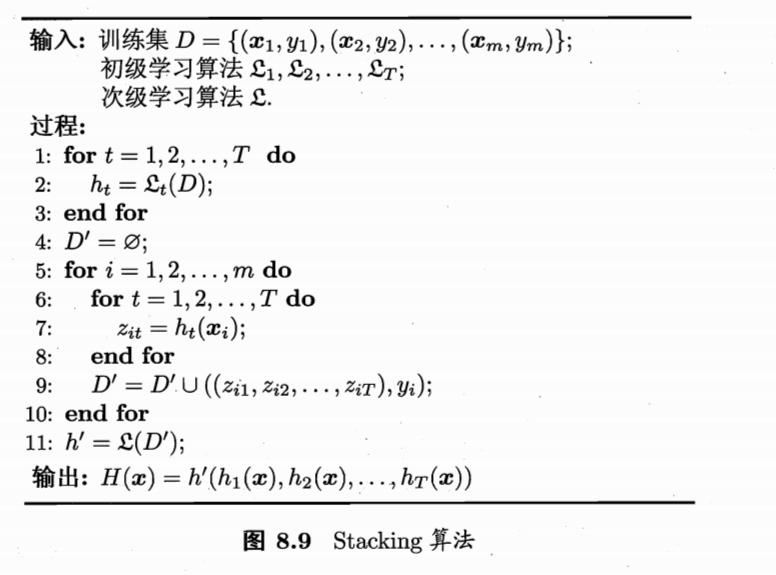Stacking的实现

1：用数据集D来训练h1,h2,h3...，

2：用这些训练出来的初级学习器在数据集D上面进行预测得到次级训练集。

3：用次级训练集来训练次级学习器。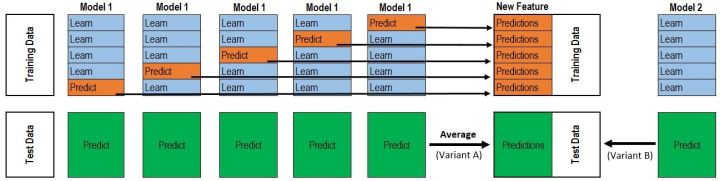import numpy as np from sklearn.model_selection import KFold def get_stacking(clf, x_train, y_train, x_test, n_folds=10): """ 这个函数是stacking的核心，使用交叉验证的方法得到次级训练集 x_train, y_train, x_test 的值应该为numpy里面的数组类型 numpy.ndarray . 如果输入为pandas的DataFrame类型则会把报错""" train_num, test_num = x_train.shape, x_test.shape second_level_train_set = np.zeros((train_num,)) second_level_test_set = np.zeros((test_num,)) test_nfolds_sets = np.zeros((test_num, n_folds)) kf = KFold(n_splits=n_folds) for i,(train_index, test_index) in enumerate(kf.split(x_train)): x_tra, y_tra = x_train[train_index], y_train[train_index] x_tst, y_tst = x_train[test_index], y_train[test_index] clf.fit(x_tra, y_tra) second_level_train_set[test_index] = clf.predict(x_tst) test_nfolds_sets[:,i] = clf.predict(x_test) second_level_test_set[:] = test_nfolds_sets.mean(axis=1) return second_level_train_set, second_level_test_set #我们这里使用5个分类算法，为了体现stacking的思想，就不加参数了 from sklearn.ensemble import (RandomForestClassifier, AdaBoostClassifier, GradientBoostingClassifier, ExtraTreesClassifier) from sklearn.svm import SVC rf_model = RandomForestClassifier() adb_model = AdaBoostClassifier() gdbc_model = GradientBoostingClassifier() et_model = ExtraTreesClassifier() svc_model = SVC() #在这里我们使用train_test_split来人为的制造一些数据 from sklearn.datasets import load_iris from sklearn.model_selection import train_test_split iris = load_iris() train_x, test_x, train_y, test_y = train_test_split(iris.data, iris.target, test_size=0.2) train_sets = [] test_sets = [] for clf in [rf_model, adb_model, gdbc_model, et_model, svc_model]: train_set, test_set = get_stacking(clf, train_x, train_y, test_x) train_sets.append(train_set) test_sets.append(test_set) meta_train = np.concatenate([result_set.reshape(-1,1) for result_set in train_sets], axis=1) meta_test = np.concatenate([y_test_set.reshape(-1,1) for y_test_set in test_sets], axis=1) #使用决策树作为我们的次级分类器 from sklearn.tree import DecisionTreeClassifier dt_model = DecisionTreeClassifier() dt_model.fit(meta_train, train_y) df_predict = dt_model.predict(meta_test) print(df_predict)

 [1 0 1 1 1 2 1 2 2 2 0 0 1 2 2 1 0 2 1 0 0 1 1 0 0 2 0 2 1 2]

 from sklearn.model_selection import KFold from sklearn.base import BaseEstimator, RegressorMixin, TransformerMixin, clone import numpy as np #对于分类问题可以使用 ClassifierMixin class StackingAveragedModels(BaseEstimator, RegressorMixin, TransformerMixin): def __init__(self, base_models, meta_model, n_folds=5): self.base_models = base_models self.meta_model = meta_model self.n_folds = n_folds # 我们将原来的模型clone出来，并且进行实现fit功能 def fit(self, X, y): self.base_models_ = [list() for x in self.base_models] self.meta_model_ = clone(self.meta_model) kfold = KFold(n_splits=self.n_folds, shuffle=True, random_state=156) #对于每个模型，使用交叉验证的方法来训练初级学习器，并且得到次级训练集 out_of_fold_predictions = np.zeros((X.shape, len(self.base_models))) for i, model in enumerate(self.base_models): for train_index, holdout_index in kfold.split(X, y): self.base_models_[i].append(instance) instance = clone(model) instance.fit(X[train_index], y[train_index]) y_pred = instance.predict(X[holdout_index]) out_of_fold_predictions[holdout_index, i] = y_pred # 使用次级训练集来训练次级学习器 self.meta_model_.fit(out_of_fold_predictions, y) return self #在上面的fit方法当中，我们已经将我们训练出来的初级学习器和次级学习器保存下来了 #predict的时候只需要用这些学习器构造我们的次级预测数据集并且进行预测就可以了 def predict(self, X): meta_features = np.column_stack([ np.column_stack([model.predict(X) for model in base_models]).mean(axis=1) for base_models in self.base_models_ ]) return self.meta_model_.predict(meta_features)369 次浏览  评价: 好 中 差订阅捐助
 相关文章 我们该如何设计数据库 数据库设计经验谈 数据库设计过程 数据库编程总结
 相关文档 数据库性能调优技巧 数据库性能调整 数据库性能优化讲座 数据库系统性能调优系列
 相关课程 高性能数据库设计与优化 高级数据库架构师 数据仓库和数据挖掘技术 Hadoop原理、部署与性能调优每天2个文档/视频 扫描微信二维码订阅
 订阅技术月刊 获得每月300个技术资源